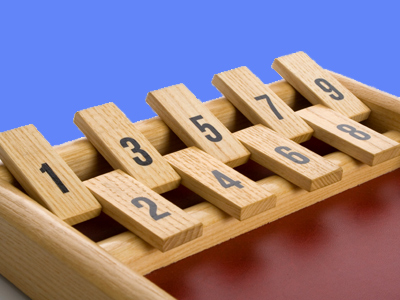Even numbers can all be divided by 2 - odd numbers cannot.

# Year 2 Numbers - Odd or Even

This quiz addresses the requirements of the National Curriculum KS1 Maths and Numeracy for children aged 6 and 7 in year 2. Specifically this quiz is aimed at the section dealing with recognising odd or even numbers.

Recognising odd or even numbers is usually a highly developed skill by Year 2. Children should be able to use odd and even when talking about the properties of number, as well as using this recognition to create and extend larger and more complex number sequences, including those using higher numbers over 100.

Question 1
If you add an even and an odd number together, the answer is always...
an even number
a number higher than 7
a number lower than 12
an odd number
For example, 5 + 4 = 9 or 15 + 10 = 25
Question 2
Even numbers can always be...
divided equally by 2
larger than odd numbers
smaller than odd numbers
divided equally by 3
Even numbers can be divided by two and give a whole number as an answer
Question 3
What are the next two numbers in this sequence: 33, 35, 37, 39
34, 36
36, 38
40, 41
41, 43
The sequence is made up of odd numbers
Question 4
Which is the next number in this sequence: 15, 17, 19, ?
18
20
21
23
The numbers are all odd, and are going up in twos
Question 5
Which set contains an odd number?
26, 88, 104, 268
54, 67, 108, 234
22, 64, 120, 238
44, 50, 82, 156
67 ends with a '7' and is odd
Question 6
Which statement is true?
Even numbers are all larger than 10
Odd numbers are sometimes in the 2 times table
Even numbers always end with 2, 4, 6, 8 or 0
Odd numbers always end with 3
Odd numbers end with 1, 3, 5, 7 or 9
Question 7
Which number isn't even?
27
36
82
90
Even numbers always end with 2, 4, 6, 8 or 0
Question 8
Which set of numbers is the odd one out?
16, 28, 36, 54
10, 30, 44, 68
13, 91, 103, 121
18, 74, 82, 104
The other sets are even, this set contains only odd numbers
Question 9
What is wrong with this sequence: 107, 109, 110, 111, 113, 115
Some numbers have a zero digit
The numbers all have 3 digits
It is too long
It contains an even number
The sequence is all odd numbers, apart from 110, which is even
Question 10
When you add two even numbers together, the result is always...
an odd number
an even number
a number higher than 10
a number less than 50
Adding two odd numbers also results in an even answer
Author:  Angela Smith

We use cookies to make your experience of our website better.

To comply with the new e-Privacy directive, we need to ask for your consent -